# Blackboard Shots with Prefix "LiBland"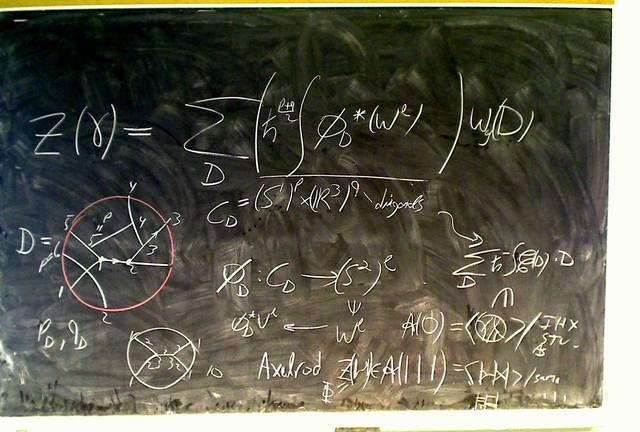121102-123510: Perturbative Chern-Simons Theory.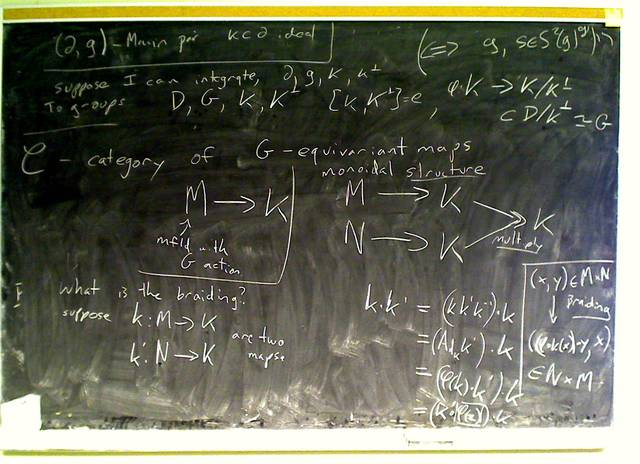111219-162602: Classical solutions of quantum Yang Baxter equations (10).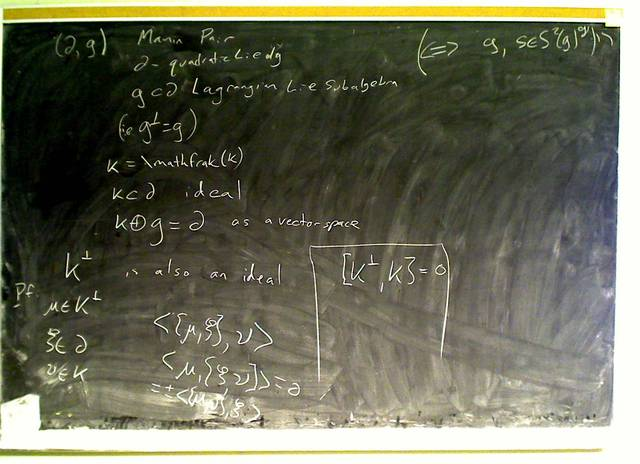111219-160255: Classical solutions of quantum Yang Baxter equations (9).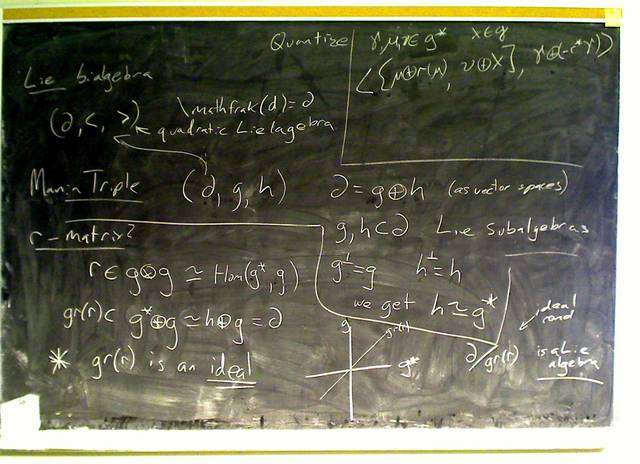111219-154526: Classical solutions of quantum Yang Baxter equations (8).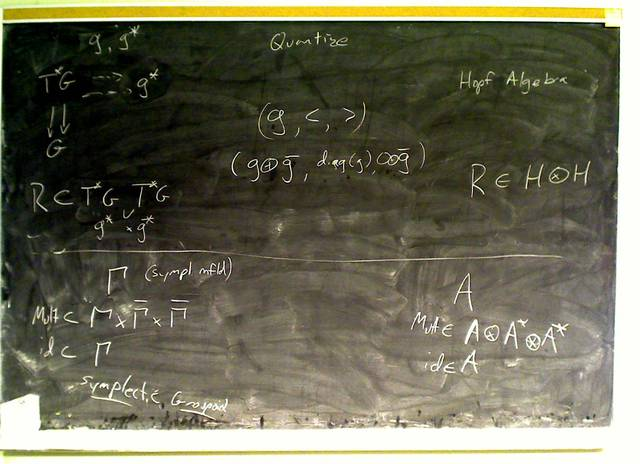111219-151359: Classical solutions of quantum Yang Baxter equations (7).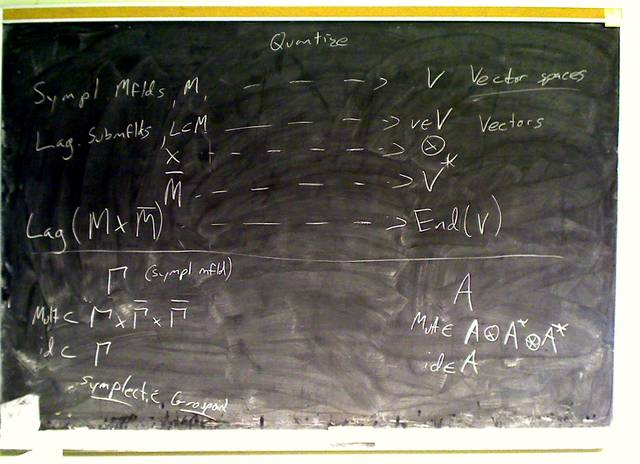111219-145703: Classical solutions of quantum Yang Baxter equations (6).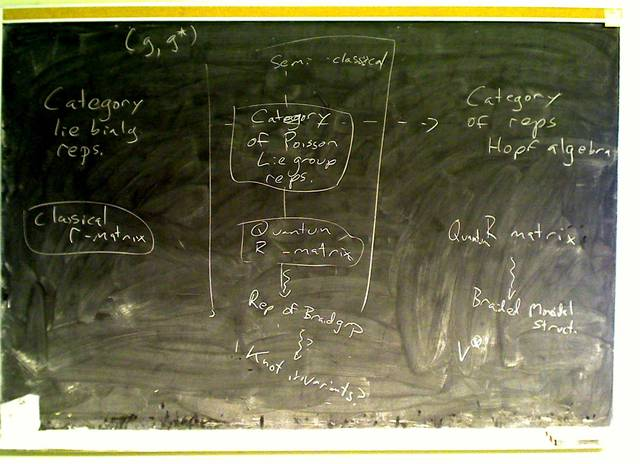111219-144812: Classical solutions of quantum Yang Baxter equations (5).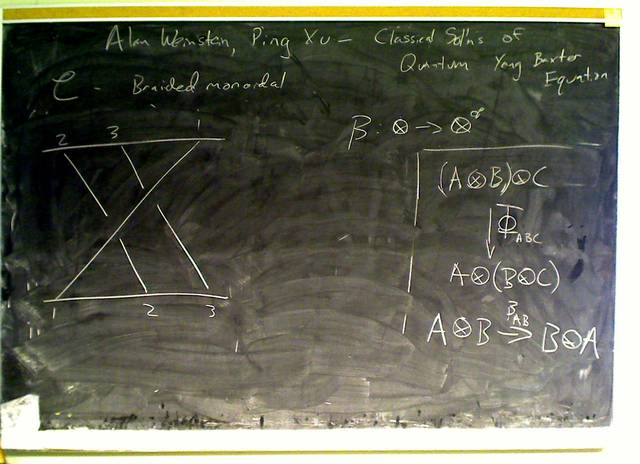111219-144214: Classical solutions of quantum Yang Baxter equations (4).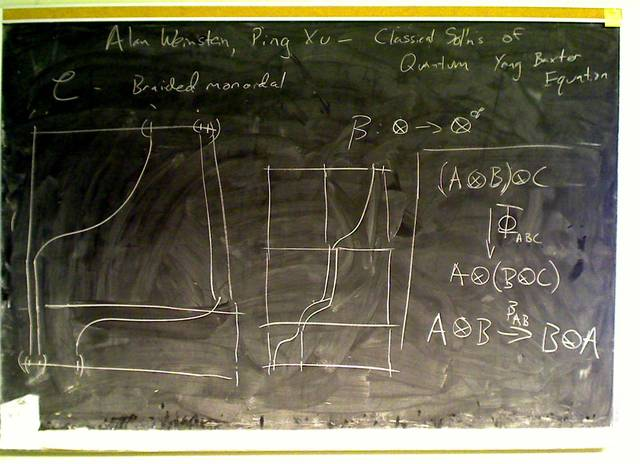111219-144053: Classical solutions of quantum Yang Baxter equations (3).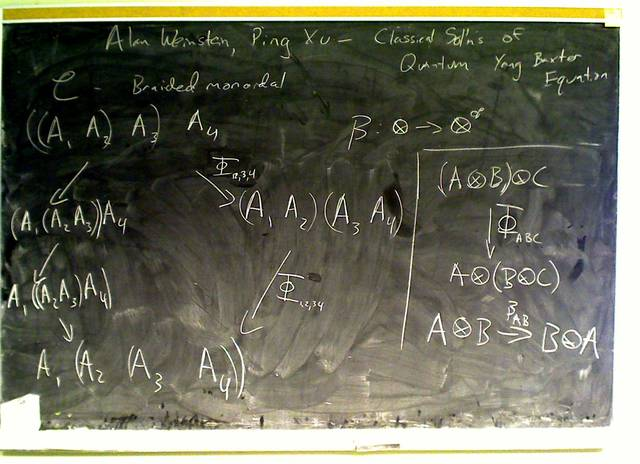111219-143809: Classical solutions of quantum Yang Baxter equations (2).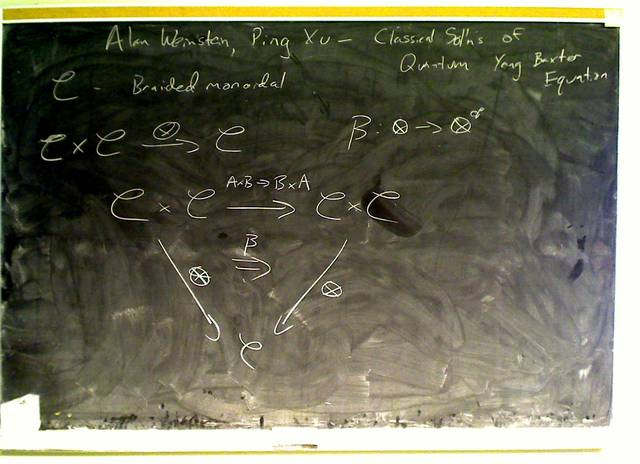111219-143310: Classical solutions of quantum Yang Baxter equations. (more...)